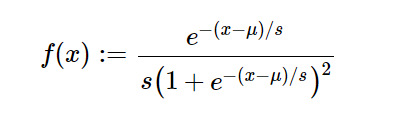# sympy.stats.Logistic() in python

With the help of `sympy.stats.Logistic()` method, we can get the continuous random variable which represents the logistic distribution.Syntax : `sympy.stats.Logistic(name, mu, s)`
Where, mu and s are real number and mu, s > 0.
Return : Return the continuous random variable.

Example #1 :
In this example we can see that by using `sympy.stats.Logistic()` method, we are able to get the continuous random variable representing logistic distribution by using this method.

 `# Import sympy and Logistic ` `from` `sympy.stats ``import` `Logistic, density ` `from` `sympy ``import` `Symbol, pprint ` ` `  `z ``=` `Symbol(``"z"``) ` `mu ``=` `Symbol(``"mu"``, positive ``=` `True``) ` `s ``=` `Symbol(``"s"``, positive ``=` `True``) ` ` `  `# Using sympy.stats.Logistic() method ` `X ``=` `Logistic(``"x"``, mu, s) ` `gfg ``=` `density(X)(z) ` ` `  `pprint(gfg) `

Output :

mu – z
——
s
e
—————-
2
/ mu – z \
| —— |
| s |
s*\e + 1/

Example #2 :

 `# Import sympy and Logistic ` `from` `sympy.stats ``import` `Logistic, density ` `from` `sympy ``import` `Symbol, pprint ` ` `  `z ``=` `0.3` `mu ``=` `5` `s ``=` `1.3` ` `  `# Using sympy.stats.Logistic() method ` `X ``=` `Logistic(``"x"``, mu, s) ` `gfg ``=` `density(X)(z) ` ` `  `pprint(gfg) `

Output :

0.0196269669241977

Attention geek! Strengthen your foundations with the Python Programming Foundation Course and learn the basics.

To begin with, your interview preparations Enhance your Data Structures concepts with the Python DS Course.

My Personal Notes arrow_drop_upCheck out this Author's contributed articles.

If you like GeeksforGeeks and would like to contribute, you can also write an article using contribute.geeksforgeeks.org or mail your article to contribute@geeksforgeeks.org. See your article appearing on the GeeksforGeeks main page and help other Geeks.

Please Improve this article if you find anything incorrect by clicking on the "Improve Article" button below.

Article Tags :

Be the First to upvote.

Please write to us at contribute@geeksforgeeks.org to report any issue with the above content.